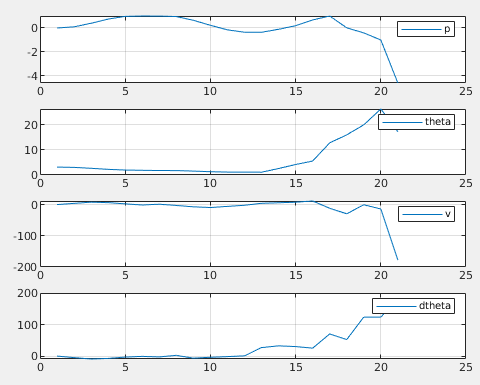# Constr_lbx wrong dimension error in MATLAB

Hi.

I am getting a Wrong Dimension error when I try to set `constr_lbx` and `constr_ubx` of the `acados_ocp` solver object.
Within the `acados_ocp_model` object I have set `Jbx` to a single row matrix with one of the columns being 1 and the rest being zero. According to the Problem definition this should give me a state constraint on just that single state controlled by `constr_lbx` and `constr_ubx`.
I am succesfully able to set that single bound value for the `acados_ocp_model` object, i.e:

``````nbx = 1;
Jbx = zeros(nbx,nx);
Jbx(1,2) = 1;
ocp_model.set('constr_Jbx', Jbx);
ocp_model.set('constr_lbx', -10);
ocp_model.set('constr_ubx', 10);
``````

But when I try to adjust the bounds dynamically during operation by setting the constraints of the `acados_ocp` object it fails:
`ocp.set('constr_lbx', -10);`
However the following works:
`ocp.set('constr_lbx', model.x0);`

So it seems like `Jbx` isn’t applied on the resulting solver object?
This aligns with the examples where `constr_lbx` is used to specify the initial condition. But if it is supposed to work with `Jbx` being specified, how would you specify the initial conditions?

Best regards
Thomas Jespersen

Hi Thomas,

``````% change values for specific shooting node using:
%   ocp.set('field', value, optional: stage_index)
ocp.set('constr_lbx', x0, 0)
``````

The intial condition is implemented as bounds on `x` on the initial stage `0`.
I think if you update your bounds stagewise for stage indices `1,.., N-1` it should work fine.

However, this

`````` ocp.set('constr_lbx', model.x0);
``````

should indeed throw an error if nbx != nx, since it tries to set this value for lbx for all stages.
I would check `ocp.model_struct.dim_nbx` and `ocp.model_struct.dim_nx` to verify they are set correctly.

Cheers,
Jonathan

Thanks, that does clarify some of it.
However if I take the `minimal_ocp_example.m` script and add the following:

``````nbx = 1;
Jbx = zeros(nbx,nx);
Jbx(1,1) = 1;
ocp_model.set('constr_Jbx', Jbx);
ocp_model.set('constr_lbx', -1)
ocp_model.set('constr_ubx', 1)
``````

This correctly puts a bound on the position state of the pendulum.
However if I want to change this bound dynamically during the loop using `ocp.set('constr_lbx', -0.5, 0)` then I get the following error:

Error using ocp_set
ocp_set: error setting constr_lbx, wrong dimension, got 1, need 4

ocp_set(obj.ext_fun_type, obj.C_ocp, obj.C_ocp_ext_fun, field, value, stage);

Error in minimal_example_ocp (line 131)
ocp.set(‘constr_lbx’, -0.5, 0)

However no error is thrown if I change the bound with the full state vector (a 4 element vector in this case):

``````% change values for specific shooting node using:
%   ocp.set('field', value, optional: stage_index)
ocp.set('constr_lbx', x0, 0)
``````

Regards Thomas

Hi Thomas,

I agree the following is not super intuitive.

The intial condition is implemented as bounds on `x` on the initial stage `0`.

So let me try to elaborate a bit more:
Internally `lbx, ubx, Jbx/idxbx` at stage `0` are used to implement the initial condition.
Since this defines the x_0 fully for an optimal control problem, the state constraints on the horizons are not enforced at the initial stage by default.
This is done by this line:

``````ocp_model.set('constr_x0', x0);
``````

A more flexible way is to set the bounds at the initial stage is setting Jbx_0, lbx_0, ubx_0, e.g.

``````ocp_model.set('constr_Jbx_0', eye(nx));
ocp_model.set('constr_lbx_0', x0);
ocp_model.set('constr_ubx_0', x0);
``````

The constraints on x, defined through `lbx, ubx, Jbx` are only enforced at the intermediate stages `1,...,N-1`.

Regarding the example:
After the modification you suggested, i.e. the following:

``````% constraints
ocp_model.set('constr_type', 'bgh');
U_max = 80;
ocp_model.set('constr_lbu', -U_max); % lower bound on u
ocp_model.set('constr_ubu', U_max);  % upper bound on u
ocp_model.set('constr_Jbu', 1);

nbx = 1;
Jbx = zeros(nbx,nx);
Jbx(1,1) = 1;
ocp_model.set('constr_Jbx', Jbx);
ocp_model.set('constr_lbx', -1)
ocp_model.set('constr_ubx', 1)

ocp_model.set('constr_x0', x0);
% ... see ocp_model.model_struct to see what other fields can be set
``````

this works to update the corresponding lower bounds on x.

``````for i = 1:N-1
ocp.set('constr_lbx', -0.5, i)
end
``````

I hope this clarifies it.

Best,
Jonathan

Indeed, that explains a lot. Thank you.
Unfortunately I still observe some oddities. If I apply the `Jbx`, `lbx` and `ubx` as mentioned above (with the limits of +/- 1) before I run the solver and then just before calling the solver I apply:

``````ocp.set('constr_lbx', -0.5, 1)
``````

Then it seems like the lower bound constraints for the other stages are disabled, since the cart position diverges towards the end and clearly exceeds the original constraint of -1.Although if I do:

``````ocp.set('constr_lbx', -0.5, 1)
ocp.set('constr_ubx', 0.5, 1)
``````

the result is different and controlled.So if you don’t mind me asking:

1. When you use `ocp_model.set('constr_Jbx', Jbx);`, `ocp_model.set('constr_lbx', -1)` and `ocp_model.set('constr_ubx', 1)` how many stages is this applied to?
2. What does `ocp.set('constr_lbx', -1)` effectively do compared to `ocp_model.set('constr_lbx', -1)` - so for both cases no stage is specified. I know from the interface code that `ocp_model.set('constr_lbx', -1)` just sets the according `model_struct` variable, but how does this propagate into setting the actual ocp compared to setting it with `ocp.set`?
3. By setting `ocp_model.set('constr_x0', x0);` does this mean that `Jbx` is set to identity for stage 0 and the `lbx` and `ubx` is set to `x0`? So this is the same as the 3 lines you wrote with e.g. `constr_Jbx_0`?
4. Can `Jbx` be defined differently and independently for each stage?

Thanks once again.

Hi Thomas,

Unfortunately, I can not reproduce the plots you posted.

I will try to answer the other questions though:

Stages 1 to N-1, stage 0 is done via `constr_x0` or `Jbx_0, lbx_0, ubx_0` as mentioned above.
For the terminal stage there are `Jbx_e, lbx_e, ubx_e`

When creating the `ocp` object, the formulation is taken from `ocp_model`. Interacting with `ocp_model` after creating `ocp`, does not influence the solver and should not be done.

Exactly.

That’s not supported by the Matlab interface.
However you could just define `Jbx` to select the component you want to constraint at some point at set trivial bounds at the non relevant stages.

Cheers